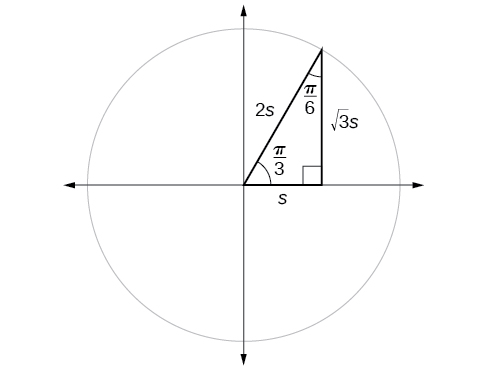# 7.2 Right triangle trigonometry  (Page 3/12)

 Page 3 / 12

Find the exact value of the trigonometric functions of $\text{\hspace{0.17em}}\frac{\pi }{4},$ using side lengths.

$\begin{array}{ccc}\hfill \text{sin}\left(\frac{\theta }{4}\right)& =& \frac{\sqrt{2}}{2},\text{cos}\left(\frac{\pi }{4}\right)=\frac{\sqrt{2}}{2},\mathrm{tan}\left(\frac{\pi }{4}\right)=1,\hfill \\ \hfill \text{sec}\left(\frac{\pi }{4}\right)& =& \sqrt{2},\text{csc}\left(\frac{\pi }{4}\right)=\sqrt{2},\text{cot}\left(\frac{\pi }{4}\right)=1\hfill \end{array}$

## Using equal cofunction of complements

If we look more closely at the relationship between the sine and cosine of the special angles, we notice a pattern. In a right triangle with angles of $\text{\hspace{0.17em}}\frac{\pi }{6}\text{\hspace{0.17em}}$ and $\text{\hspace{0.17em}}\frac{\pi }{3},$ we see that the sine of $\text{\hspace{0.17em}}\frac{\pi }{3},$ namely $\text{\hspace{0.17em}}\frac{\sqrt{3}}{2},$ is also the cosine of $\text{\hspace{0.17em}}\frac{\pi }{6},$ while the sine of $\text{\hspace{0.17em}}\frac{\pi }{6},$ namely $\text{\hspace{0.17em}}\frac{1}{2},$ is also the cosine of $\text{\hspace{0.17em}}\frac{\pi }{3}.$

$\begin{array}{cccc}\hfill \mathrm{sin}\frac{\pi }{3}& =\mathrm{cos}\frac{\pi }{6}\hfill & =\frac{\sqrt{3}s}{2s}\hfill & =\frac{\sqrt{3}}{2}\hfill \\ \hfill \mathrm{sin}\frac{\pi }{6}& =\mathrm{cos}\frac{\pi }{3}\hfill & =\frac{s}{2s}\hfill & =\frac{1}{2}\hfill \end{array}$The sine of   π 3   equals the cosine of   π 6   and vice versa.

This result should not be surprising because, as we see from [link] , the side opposite the angle of $\text{\hspace{0.17em}}\frac{\pi }{3}\text{\hspace{0.17em}}$ is also the side adjacent to $\text{\hspace{0.17em}}\frac{\pi }{6},$ so $\text{\hspace{0.17em}}\mathrm{sin}\left(\frac{\pi }{3}\right)\text{\hspace{0.17em}}$ and $\text{\hspace{0.17em}}\mathrm{cos}\left(\frac{\pi }{6}\right)\text{\hspace{0.17em}}$ are exactly the same ratio of the same two sides, $\text{\hspace{0.17em}}\sqrt{3}s\text{\hspace{0.17em}}$ and $\text{\hspace{0.17em}}2s.\text{\hspace{0.17em}}$ Similarly, $\text{\hspace{0.17em}}\mathrm{cos}\left(\frac{\pi }{3}\right)\text{\hspace{0.17em}}$ and $\text{\hspace{0.17em}}\mathrm{sin}\left(\frac{\pi }{6}\right)\text{\hspace{0.17em}}$ are also the same ratio using the same two sides, $\text{\hspace{0.17em}}s\text{\hspace{0.17em}}$ and $\text{\hspace{0.17em}}2s.$

The interrelationship between the sines and cosines of $\text{\hspace{0.17em}}\frac{\pi }{6}\text{\hspace{0.17em}}$ and $\text{\hspace{0.17em}}\frac{\pi }{3}\text{\hspace{0.17em}}$ also holds for the two acute angles in any right triangle, since in every case, the ratio of the same two sides would constitute the sine of one angle and the cosine of the other. Since the three angles of a triangle add to $\text{\hspace{0.17em}}\pi ,$ and the right angle is $\text{\hspace{0.17em}}\frac{\pi }{2},$ the remaining two angles must also add up to $\text{\hspace{0.17em}}\frac{\pi }{2}.\text{\hspace{0.17em}}$ That means that a right triangle can be formed with any two angles that add to $\text{\hspace{0.17em}}\frac{\pi }{2}\text{\hspace{0.17em}}$ —in other words, any two complementary angles. So we may state a cofunction identity : If any two angles are complementary, the sine of one is the cosine of the other, and vice versa. This identity is illustrated in [link] .

Using this identity, we can state without calculating, for instance, that the sine of $\text{\hspace{0.17em}}\frac{\pi }{12}\text{\hspace{0.17em}}$ equals the cosine of $\text{\hspace{0.17em}}\frac{5\pi }{12},$ and that the sine of $\text{\hspace{0.17em}}\frac{5\pi }{12}\text{\hspace{0.17em}}$ equals the cosine of $\text{\hspace{0.17em}}\frac{\pi }{12}.\text{\hspace{0.17em}}$ We can also state that if, for a given angle then $\text{\hspace{0.17em}}\mathrm{sin}\left(\frac{\pi }{2}-t\right)=\frac{5}{13}\text{\hspace{0.17em}}$ as well.

## Cofunction identities

 $\mathrm{cos}\text{\hspace{0.17em}}t=\mathrm{sin}\left(\frac{\pi }{2}-t\right)$ $\mathrm{sin}\text{\hspace{0.17em}}t=\mathrm{cos}\left(\frac{\pi }{2}-t\right)$ $\mathrm{tan}\text{\hspace{0.17em}}t=\mathrm{cot}\left(\frac{\pi }{2}-t\right)$ $\mathrm{cot}\text{\hspace{0.17em}}t=\mathrm{tan}\left(\frac{\pi }{2}-t\right)$ $\mathrm{sec}\text{\hspace{0.17em}}t=\mathrm{csc}\left(\frac{\pi }{2}-t\right)$ $\mathrm{csc}\text{\hspace{0.17em}}t=\mathrm{sec}\left(\frac{\pi }{2}-t\right)$

Given the sine and cosine of an angle, find the sine or cosine of its complement.

1. To find the sine of the complementary angle, find the cosine of the original angle.
2. To find the cosine of the complementary angle, find the sine of the original angle.

## Using cofunction identities

If $\text{\hspace{0.17em}}\mathrm{sin}\text{\hspace{0.17em}}t=\frac{5}{12},$ find $\text{\hspace{0.17em}}\mathrm{cos}\left(\frac{\pi }{2}-t\right).$

According to the cofunction identities for sine and cosine, we have the following.

$\mathrm{sin}\text{\hspace{0.17em}}t=\mathrm{cos}\text{\hspace{0.17em}}\left(\frac{\pi }{2}-t\right)$

So

$\mathrm{cos}\text{\hspace{0.17em}}\left(\frac{\pi }{2}-t\right)=\frac{5}{12}$

If $\text{\hspace{0.17em}}\mathrm{csc}\text{\hspace{0.17em}}\left(\frac{\pi }{6}\right)=2,$ find $\text{\hspace{0.17em}}\mathrm{sec}\text{\hspace{0.17em}}\left(\frac{\pi }{3}\right).$

2

## Using trigonometric functions

In previous examples, we evaluated the sine and cosine in triangles where we knew all three sides. But the real power of right-triangle trigonometry emerges when we look at triangles in which we know an angle but do not know all the sides.

Given a right triangle, the length of one side, and the measure of one acute angle, find the remaining sides.

1. For each side, select the trigonometric function that has the unknown side as either the numerator or the denominator. The known side will in turn be the denominator or the numerator.
2. Write an equation setting the function value of the known angle equal to the ratio of the corresponding sides.
3. Using the value of the trigonometric function and the known side length, solve for the missing side length.

what is the function of sine with respect of cosine , graphically
tangent bruh
Steve
cosx.cos2x.cos4x.cos8x
sinx sin2x is linearly dependent
what is a reciprocal
The reciprocal of a number is 1 divided by a number. eg the reciprocal of 10 is 1/10 which is 0.1
Shemmy
Reciprocal is a pair of numbers that, when multiplied together, equal to 1. Example; the reciprocal of 3 is ⅓, because 3 multiplied by ⅓ is equal to 1
Jeza
each term in a sequence below is five times the previous term what is the eighth term in the sequence
I don't understand how radicals works pls
How look for the general solution of a trig function
stock therom F=(x2+y2) i-2xy J jaha x=a y=o y=b
sinx sin2x is linearly dependent
cr
root under 3-root under 2 by 5 y square
The sum of the first n terms of a certain series is 2^n-1, Show that , this series is Geometric and Find the formula of the n^th
cosA\1+sinA=secA-tanA
Wrong question
why two x + seven is equal to nineteen.
The numbers cannot be combined with the x
Othman
2x + 7 =19
humberto
2x +7=19. 2x=19 - 7 2x=12 x=6
Yvonne
because x is 6
SAIDI
what is the best practice that will address the issue on this topic? anyone who can help me. i'm working on my action research.
simplify each radical by removing as many factors as possible (a) √75
how is infinity bidder from undefined?ByByBy OpenStaxBy Robert MorrisBy OpenStaxBy Jazzycazz JacksonBy Megan EarhartBy Sandhills MLTBy Stephen VoronBy OpenStaxBy OpenStaxBy Michael Nelson